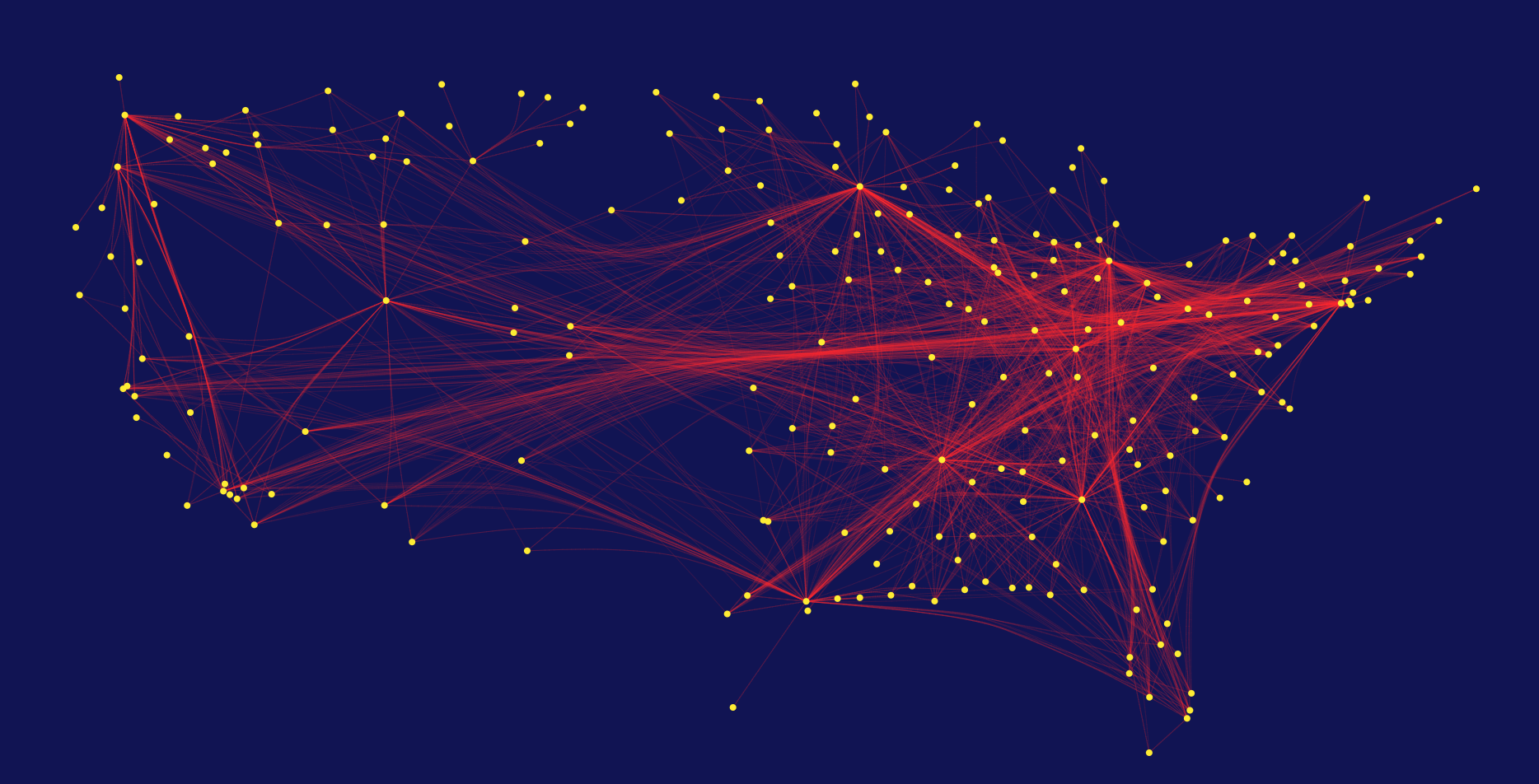# Simulating Particles in D3Mapping the interaction between particles is a common problem in Physics, but it can be hard to visualize . D3 is a tool that can help solve this issue by simulating particles in a simple way.

Here’s how D3 works.

# The Physics behind D3

D3 (Data-Driven Documents) is a JavaScript library for visualizing data using SVG, Canvas, and HTML. D3 creates all kinds of visualizations, from the typical chart bars to maps. To simulate particles we have to focus on the force-directed graph layout. The module is called d3-force. With this, you can do amazing things like this example or projects like this game about epidemic prevention.

This module uses the “Verlet Integration”  numerical method, which integrates Newton’s equations of motion.

The integral of acceleration is always velocity v(t), whose integral is always position, x(t). If we know the initial position (x_0), and velocity as well as the infinite number of derivatives, and then we integrated each one to get their contribution to position, we could know the position at any time,

Now if we take this equation both forward in time and backwards in time by some small Δt so the equations would look like,

and if you add them together,

after simplifying the equation you get,

You can compute the position in the future just by knowing the previous position and the acceleration. There are higher derivatives like jounce but when dealing with classical mechanics there are zero forces associated with derivatives higher than acceleration so the term O can be omitted.

To make calculations easier, D3 makes some assumptions,

• constant unit time step Δt = 1 for each step
• constant unit mass m = 1 for all particles

Considering that F=ma, the force will have the value of just the acceleration. Since we are taking Δt=1s we can have the velocity each step in time and then the position of the particle.

You can simulate the action of any force in multiple particles by assuming that the interaction between one partícule and the others does not affect the interaction of the whole system, this is called the superposition principle.

# Particles and Code

D3 has multiple types of forces: charge, link, positioning, collision, etc.  If you pick link force you can simulate not only particles, but more complex structures like molecules. Let’s stay with that one.

In this type of force, you have particles interacting with other particles by a “stick” that binds them to each other.

You have to define the nodes and the particles that are joined to them.

Now, D3 updates the position in some time interval (Δt=1) by just adding the velocity. The library takes into account that the distance between them has to be the same.

In the code above, we have two loops. First, you have to do the calculations for each node (in this case called link) and then for each particle that it is bound to.

Each link has its source and target node, and each node has a x and y position (target.x target.y source.x source.y)  and it’s x and y velocity (target.vx target.vy source.vx source.vy) . It also has its strengths associated to the stick that will modify the behavior of the distances between the nodes (l).

There’s much more you can do with D3, besides adding and subtracting velocities, like combining different types of forces, and even interact with the particles.

And here is a simple example using charge and  link force, try it!

# Finally…

D3 is a powerful tool to create interactive data visualizations, and even games. It can be used in any professional setting, or even for school projects (I used it a couple of times).

If you have any questions send me a line at [email protected]

References:

Focus Mode

## Contact Request

We will call you right away. All information is kept private